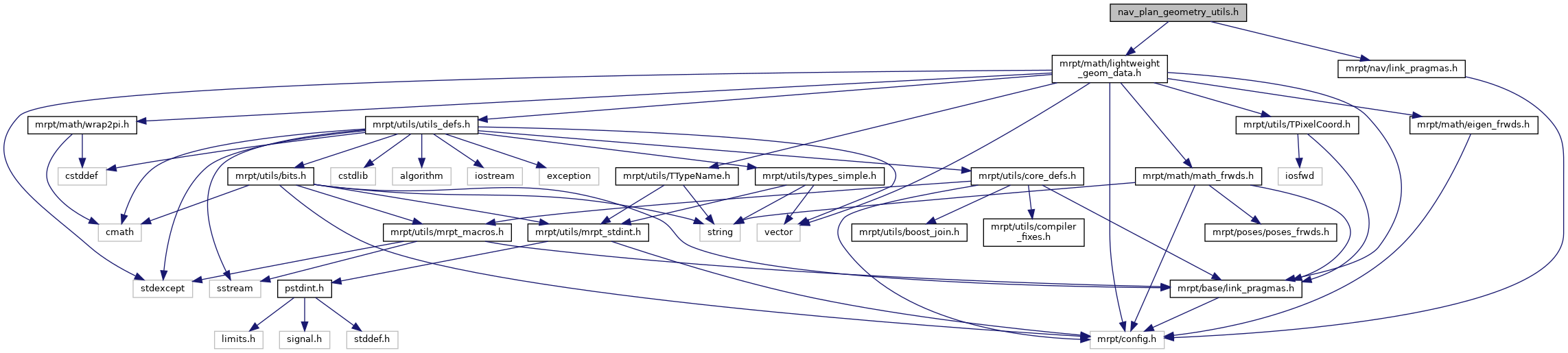Main MRPT website > C++ reference for MRPT 1.5.7
nav_plan_geometry_utils.h File Reference
`#include <mrpt/math/lightweight_geom_data.h>`
`#include <mrpt/nav/link_pragmas.h>`
Include dependency graph for nav_plan_geometry_utils.h:This graph shows which files directly or indirectly include this file:Go to the source code of this file.

## Namespaces

mrpt
This is the global namespace for all Mobile Robot Programming Toolkit (MRPT) libraries.

mrpt::nav

## Functions

bool NAV_IMPEXP mrpt::nav::collision_free_dist_segment_circ_robot (const mrpt::math::TPoint2D &p_start, const mrpt::math::TPoint2D &p_end, const double robot_radius, const mrpt::math::TPoint2D &obstacle, double &out_col_dist)
Computes the collision-free distance for a linear segment path between two points, for a circular robot, and a point obstacle (ox,oy). More...

bool NAV_IMPEXP mrpt::nav::collision_free_dist_arc_circ_robot (const double arc_radius, const double robot_radius, const mrpt::math::TPoint2D &obstacle, double &out_col_dist)
Computes the collision-free distance for a forward path (+X) circular arc path segment from pose (0,0,0) and radius of curvature R (>0 -> +Y, <0 -> -Y), a circular robot and a point obstacle (ox,oy). More...

 Page generated by Doxygen 1.8.14 for MRPT 1.5.7 Git: 8277875f6 Mon Jun 11 02:47:32 2018 +0200 at lun oct 28 01:50:49 CET 2019